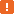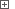# Accessing Rows, Columns and Cells in Syncfusion GridControlIn order to perform various operations over Syncfusion's GridControl, you need to obtain the objects corresponding to grid rows, columns and cells. This topic describes the properties and methods of the GridControl object that can be used for this purpose.To perform these actions, TestComplete should have access to internal objects, properties and methods of the GridControl object. For this purpose, the .NET Application Support plugin must be installed and enabled.When testing Syncfusion GridControl controls, use specific methods and properties of the corresponding `SyncfusionEssGrid` object. You can call these methods and properties from your keyword tests, as well as from scripts. This topic describes how to work with an object’s properties and methods from your scripts. However, when testing a GridControl control from your keyword test, you can use the same methods and properties calling them from keyword test operations. For more information, see Keyword Tests Basic Operations.
##### Identifying Rows and Columns

The rows and columns in the GridControl are identified by their indexes. The numerations are zero-based, so the first row and column have an index of 0, the second - 1, and so on. The index of the last grid row is `Model.RowCount` and the last column is `Model.ColCount`. So, the total number of grid rows is `Model.RowCount`+1 and for columns, `Model.ColCount`+1.

Note that the rows and columns numeration includes row and column headers. That is, the starting indexes correspond to grid headers and indexes of the actual data rows and columns start from 1 or a greater number, depending on the number of headers. If you need to locate the range of actual data cells, use the grid’s `GridCellsRange` property. The `Top`, `Bottom`, `Left` and `Right` properties of the returned object let you retrieve bound indexes of grid rows and columns that contain data cells. To determine the number of data rows and columns, use the `GridCellsRange.Height` and `GridCellsRange.Width` properties, respectively.

Below is a code snippet that posts information about the GridControl to the test log:

JavaScript, JScript

function Test ()
{
var p, Grid;

// Obtain the application process and the grid object
p = Sys.Process("GridControlSort");
Grid = p.WinFormsObject("Form1").WinFormsObject("gridControl1");

// Post information about the grid structure to the test log
Log.Message ("Total number of rows: " + (Grid.Model.RowCount+1));
Log.Message ("Total number of columns: " + (Grid.Model.ColCount+1));
Log.Message ("Number of data rows: " + Grid.GridCellsRange.Height);
Log.Message ("Number of data columns: " + Grid.GridCellsRange.Width);
Log.Message ("Index of the first data row: " + Grid.GridCellsRange.Top);
Log.Message ("Index of the first data column: " + Grid.GridCellsRange.Left);
}

Python

``````def Test ():

# Obtain the application process and the grid object
p = Sys.Process("GridControlSort")
Grid = p.WinFormsObject("Form1").WinFormsObject("gridControl1")

# Post information about the grid structure to the test log
Log.Message ("Total number of rows: " + (Grid.Model.RowCount+1))
Log.Message ("Total number of columns: " + (Grid.Model.ColCount+1))
Log.Message ("Number of data rows: " + Grid.GridCellsRange.Height)
Log.Message ("Number of data columns: " + Grid.GridCellsRange.Width)
Log.Message ("Index of the first data row: " + Grid.GridCellsRange.Top)
Log.Message ("Index of the first data column: " + Grid.GridCellsRange.Left)``````

VBScript

Sub Test
Dim p, Grid

' Obtain the application process and the grid object
Set p = Sys.Process("GridControlSort")
Set Grid = p.WinFormsObject("Form1").WinFormsObject("gridControl1")

' Post information about the grid structure to the test log
Call Log.Message ("Total number of rows: " & (Grid.Model.RowCount+1))
Call Log.Message ("Total number of columns: " & (Grid.Model.ColCount+1))
Call Log.Message ("Number of data rows: " & Grid.GridCellsRange.Height)
Call Log.Message ("Number of data columns: " & Grid.GridCellsRange.Width)
Call Log.Message ("Index of the first data row: " & Grid.GridCellsRange.Top)
Call Log.Message ("Index of the first data column: " & Grid.GridCellsRange.Left)
End Sub

DelphiScript

procedure Test;
var p, Grid : OleVariant;
begin
// Obtain the application process and the grid object
p := Sys.Process('GridControlSort');
Grid := p.WinFormsObject('Form1').WinFormsObject('gridControl1');

// Post information about the grid structure to the test log
Log.Message ('Total number of rows: ' + aqConvert.VarToStr(Grid.Model.RowCount+1));
Log.Message ('Total number of columns: ' + aqConvert.VarToStr(Grid.Model.ColCount+1));
Log.Message ('Number of data rows: ' + aqConvert.VarToStr(Grid.GridCellsRange.Height));
Log.Message ('Number of data columns: ' + aqConvert.VarToStr(Grid.GridCellsRange.Width));
Log.Message ('Index of the first data row: ' + aqConvert.VarToStr(Grid.GridCellsRange.Top));
Log.Message ('Index of the first data column: ' + aqConvert.VarToStr(Grid.GridCellsRange.Left));
end;

C++Script, C#Script

function Test ()
{
var p, Grid;

// Obtain the application process and the grid object
p = Sys["Process"]("GridControlSort");
Grid = p["WinFormsObject"]("Form1")["WinFormsObject"]("gridControl1");

// Post information about the grid structure to the test log
Log["Message"]("Total number of rows: " + (Grid["Model"]["RowCount"]+1));
Log["Message"]("Total number of columns: " + (Grid["Model"]["ColCount"]+1));
Log["Message"]("Number of data rows: " + Grid["GridCellsRange"]["Height"]);
Log["Message"]("Number of data columns: " + Grid["GridCellsRange"]["Width"]);
Log["Message"]("Index of the first data row: " + Grid["GridCellsRange"]["Top"]);
Log["Message"]("Index of the first data column: " + Grid["GridCellsRange"]["Left"]);
}

##### Accessing Cells

You can access a particular GridControl cell using the grid’s `Item` property. This property takes the desired cell’s row and column indexes as parameters:

GridObj.Item (RowIndex, ColIndex)

To obtain information about the currently selected cell, use the grid’s `CurrentCell` property. The `CurrentCell.RowIndex` and `CurrentCell.ColIndex` properties lets you determine indexes of the current row and column, respectively.

The code snippet below demonstrates how you can get information about the currently selected grid cell:

###### ExampleView description

JavaScript, JScript

function Main ()
{
var p, Grid, Cell;

// Obtain the application process and the grid object
p = Sys.Process("GridControlSort");
Grid = p.WinFormsObject("Form1").WinFormsObject("gridControl1");

// Click a grid cell
ClickCell (Grid, 11, 2);

// Get information about the selected cell
Cell = Grid.CurrentCell;
Log.Message ("Current row index: " + Cell.RowIndex);
Log.Message ("Current column index: " + Cell.ColIndex);
Log.Message ("Current cell text: " + Grid.Item(Cell.RowIndex, Cell.ColIndex).FormattedText.OleValue);
}

function ClickCell (Grid, RowIndex, ColIndex)
{
// Make cell visible
Grid.ScrollCellInView_3 (RowIndex, ColIndex);

// Get the cell coordinates
var rect = Grid.RangeInfoToRectangle (Grid.GridCellsRange.Cell(RowIndex, ColIndex));

Grid.Click (rect.X + rect.Width/2, rect.Y + rect.Height/2);
}

Python

``````def Main ():

# Obtain the application process and the grid object
p = Sys.Process("GridControlSort")
Grid = p.WinFormsObject("Form1").WinFormsObject("gridControl1")

# Click a grid cell
ClickCell (Grid, 11, 2)

# Get information about the selected cell
Cell = Grid.CurrentCell
Log.Message ("Current row index: " + Cell.RowIndex)
Log.Message ("Current column index: " + Cell.ColIndex)
Log.Message ("Current cell text: " + Grid.Item(Cell.RowIndex, Cell.ColIndex).FormattedText.OleValue)

def ClickCell (Grid, RowIndex, ColIndex):
# Make cell visible
Grid.ScrollCellInView_3 (RowIndex, ColIndex)

# Get the cell coordinates
rect = Grid.RangeInfoToRectangle (Grid.GridCellsRange.Cell[RowIndex, ColIndex])

Grid.Click (rect.X + rect.Width/2, rect.Y + rect.Height/2)``````

VBScript

Sub Main
Dim p, Grid, Cell

' Obtain the application process and the grid object
Set p = Sys.Process("GridControlSort")
Set Grid = p.WinFormsObject("Form1").WinFormsObject("gridControl1")

' Click a grid cell
Call ClickCell (Grid, 11, 2)

' Get information about the selected cell
Set Cell = Grid.CurrentCell
Log.Message ("Current row index: " & Cell.RowIndex)
Log.Message ("Current column index: " & Cell.ColIndex)
Log.Message ("Current cell text: " & Grid.Item(Cell.RowIndex, Cell.ColIndex).FormattedText.OleValue)
End Sub

Sub ClickCell (Grid, RowIndex, ColIndex)
Dim rect

' Make cell visible
Call Grid.ScrollCellInView_3 (RowIndex, ColIndex)

' Get the cell coordinates
Set rect = Grid.RangeInfoToRectangle (Grid.GridCellsRange.Cell(RowIndex, ColIndex))

Call Grid.Click (rect.X + rect.Width/2, rect.Y + rect.Height/2)
End Sub

DelphiScript

procedure ClickCell (Grid, RowIndex, ColIndex);
var rect : OleVariant;
begin
// Make cell visible
Grid.ScrollCellInView_3 (RowIndex, ColIndex);

// Get the cell coordinates
rect := Grid.RangeInfoToRectangle (Grid.GridCellsRange.Cell(RowIndex, ColIndex));

Grid.Click (rect.X + rect.Width/2, rect.Y + rect.Height/2);
end;

procedure Main;
var p, Grid, Cell : OleVariant;
begin
// Obtain the application process and the grid object
p := Sys.Process('GridControlSort');
Grid := p.WinFormsObject('Form1').WinFormsObject('gridControl1');

// Click a grid cell
ClickCell (Grid, 11, 2);

// Get information about the selected cell
Cell := Grid.CurrentCell;
Log.Message ('Current row index: ' + aqConvert.VarToStr(Cell.RowIndex));
Log.Message ('Current column index: ' + aqConvert.VarToStr(Cell.ColIndex));
Log.Message ('Current cell text: ' + Grid.Item[Cell.RowIndex, Cell.ColIndex].FormattedText.OleValue);
end;

C++Script, C#Script

function Main ()
{
var p, Grid, Cell;

// Obtain the application process and the grid object
p = Sys["Process"]("GridControlSort");
Grid = p["WinFormsObject"]("Form1")["WinFormsObject"]("gridControl1");

// Click a grid cell
ClickCell (Grid, 11, 2);

// Get information about the selected cell
Cell = Grid["CurrentCell"];
Log["Message"]("Current row index: " + Cell["RowIndex"]);
Log["Message"]("Current column index: " + Cell["ColIndex"]);
Log["Message"]("Current cell text: " + Grid["Item"](Cell["RowIndex"], Cell["ColIndex"])["FormattedText"]["OleValue"]);
}

function ClickCell (Grid, RowIndex, ColIndex)
{
// Make cell visible
Grid["ScrollCellInView_3"](RowIndex, ColIndex);

// Get the cell coordinates
var rect = Grid["RangeInfoToRectangle"](Grid["GridCellsRange"]["Cell"](RowIndex, ColIndex));

Grid["Click"](rect["X"] + rect["Width"]/2, rect["Y"] + rect["Height"]/2);
}